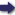(London :  Kegan Paul, Trench, Trübner & Co.,  1910.)

 Tools

## Search this bookPrev Page 119 Next``` CHAPTER LXI. 119 We have already, in former chapters, described the dominants of the hours, of the mithiXrtas, of the halves of the lunar days, of the single days in the white and black halves of the month, of the parvans of the eclipses, and of the single manvctntarcts. What there is more of the same kind we shall give in this place. In computing the dominant of the yectr, the Hindus computa- use another method than the Western nations, who dominant of compute it, according to certain well-known rules, from accordtng to .1 7 1 p rm -1 • . f the Khanda. the ascendens or horoscope of a year. The dominant of khadyaka. the year as well as the dominant of the month are the rulers of certain periodically recurring parts of time, and are by a certain calculation derived from the domi¬ nants of the hours and the dominants of the days. If you want to find the dominant of the year, com¬ pute the sum of days of the date in question according to the rules of the canon Khanclctkhddyaka, which is the most universally used among them. Subtract there¬ from 2201, and divide the remainder by 360. Multiply the quotient by 3, and add to the product always 3. Divide the sum by 7. The remainder, a number under 7, you count off on the week-days, beginning with Sunday. The dominant of that day you come to is at the same time the dominant of the year. The remainders you get by the division are the days of his rule which have already elapsed. These, together with the days of his rule which have not yet elapsed, give the sum of 360. It is the same whether we reckon as we have just explained, or add to the here-mentioned sum of days 319, instead of subtracting from it. If you want to find the dominant of the month, sub- iiow to find tract 71 from the sum of days of the date in question, nantofthe and divide the remainder by 30. Double the quotient and add i. The sum divide by 7, and the remainder count off on the week-days, beginning with Sunday. The dominant of the day you come to is at the same ```Prev Page 119 Next